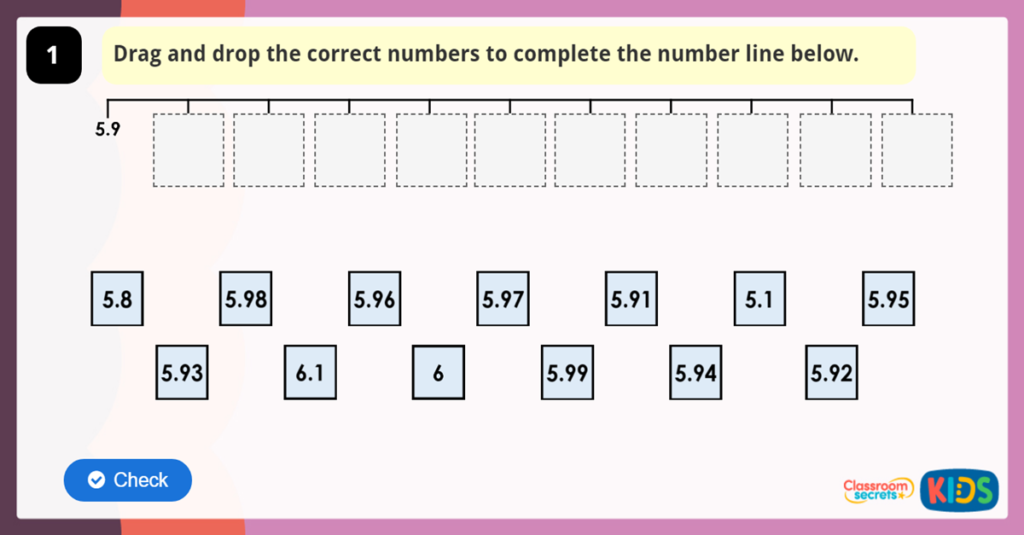To make finding the White Rose Resources easier we have created a dedicated page for you to help your child with Maths at home.

Overview

Week 1 (week beginning 20/04/20):Year 4 Decimals Knowledge Organiser

Lesson 1 – Making A Whole

Lesson 2 – Write Decimals

Lesson 3 – Compare Decimals

Lesson 4 – Order Decimals

Week 2 (week beginning 27/04/20):

Lesson 1 – Round Decimals

Lesson 2 – Halves and Quarters

Lesson 3 – Pounds and Pence

Lesson 4 – Ordering Money

Week 3 (week beginning 04/05/20):Year 4 Multiplication and Division Knowledge Organiser

Lesson 1 – Multiply 2 Digits by 1 Digit

Lesson 2 – Multiply 3 Digits by 1 Digit

Lesson 3 – Divide 2 Digits by 1 Digit

Lesson 4 – Divide 3 Digits by 1 Digit

Week 4 (week beginning 11/05/20):

Year 4 Area and Perimeter Knowledge Organiser

Lesson 1 – Correspondence Problems

Lesson 2 – Perimeter Of A Rectangle

Lesson 3 –  Perimeter Of Rectilinear Shapes

Lesson 4 – Counting Squares

Week 5 (week beginning 18/05/20):Year 4 Fractions Knowledge Organiser

Lesson 1 – Recognise Tenths and Hundredths

Lesson 2 – Equivalent Fractions

Lesson 3 – Equivalent Fractions

Lesson 4 – Fractions Greater Than 1

Week 5 Alternative (week beginning 18/05/20):

Alternative Plan

Lesson 1 – What Is A Fraction?

Week 6 (week beginning 01/06/20):Lesson 1 – Add 2 or More Fractions

Lesson 2 – Subtract 2 Fractions

Lesson 3 – Fractions Of A Quantity

Lesson 4 – Calculate Quantities

Week 7 (week beginning 08/06/20):

Lesson 1 - Tenths As Decimals

Lesson 2 - Dividing 1 Digit By 10

Lesson 3 - Hundredths As Decimals

Lesson 4 - Dividing 1 and 2 Digits by 100

Week 7 Alternative (week beginning 08/06/20):

Alternative Plan

Lesson 1 - 1s, 10s, 100s, 1000s

Lesson 2 – Add 2 Digit Numbers (No Exchange)

Lesson 3 – Add Two 4 Digit Numbers (One Exchange)

Lesson 4 – Add Two 4 Digit Numbers (More Than One Exchange)

Week 8 (week beginning 15/06/20):Lesson 1 - Write Decimals

Lesson 2 – Compare Decimals

Lesson 3 – Order Decimals

Lesson 4 – Round Decimals

Week 8 Alternative (week beginning 15/06/20):

Alternative Plan

Lesson 1 – Subtract Two 4 Digit Numbers (No Exchange)

Lesson 2 – Subtract Two 4 Digit Numbers (One Exchange)

Lesson 3 – Subtract Two 4 Digit Numbers (More Than One Exchange)

Lesson 4 – Efficient Subtraction

Week 9 (week beginning 22/06/20):Lesson 1 – Pounds and Pence

Lesson 2 – Ordering Money

Lesson 3 – Estimating Money

Lesson 4 – Four Operations

Week 9 Alternative (week beginning 22/06/20):

Alternative Plan

Lesson 2 – Checking Strategies

Week 10 (week beginning 29/06/20):Year 4 Statistics Knowledge Organiser

Lesson 1 – Interpret Charts

Lesson 2 – Comparison, Sum and Difference

Lesson 3 – Introducing Line Graphs

Lesson 4 – Line Graphs

Week 11 (week beginning 06/07/20):

Lesson 1 – Identify Angles

Lesson 2 – Compare and Order Angles

Lesson 3 – Triangles

Week 12 (week beginning 13/07/20):

Lesson 1 – Lines Of Symmetry

Lesson 2 – Complete A Symmetric Figure